Ex 9.4

Chapter 9 Class 12 Differential Equations
Serial order wise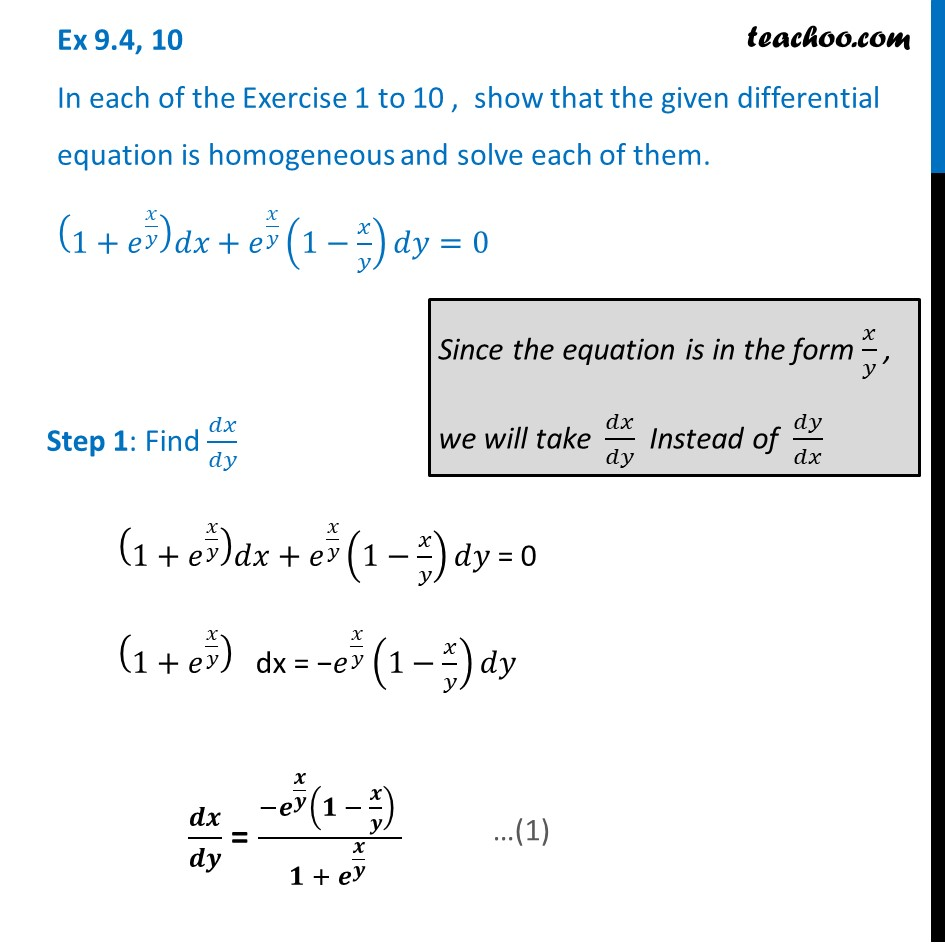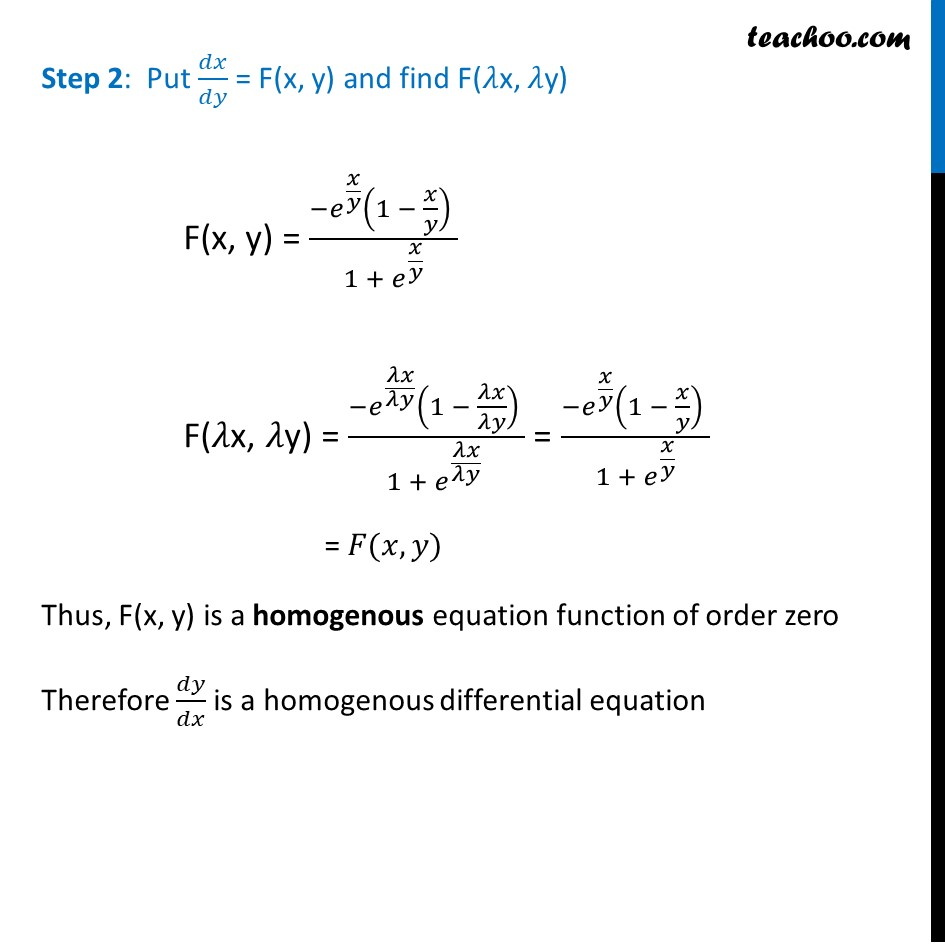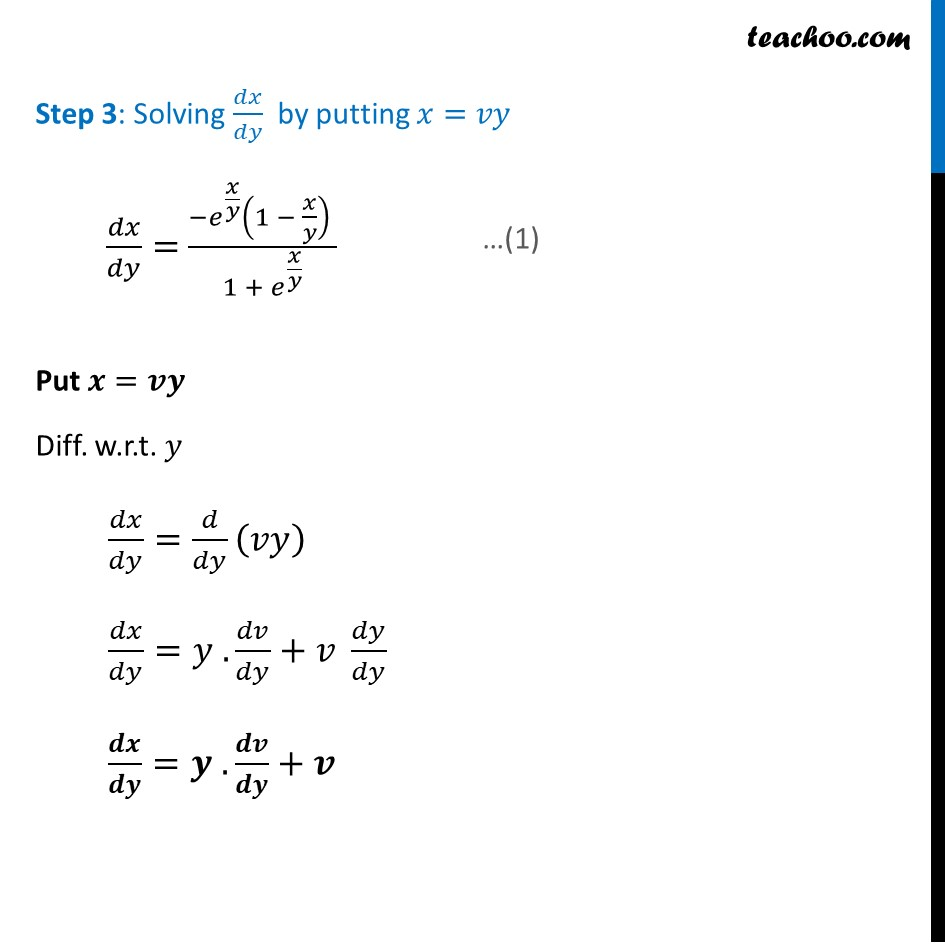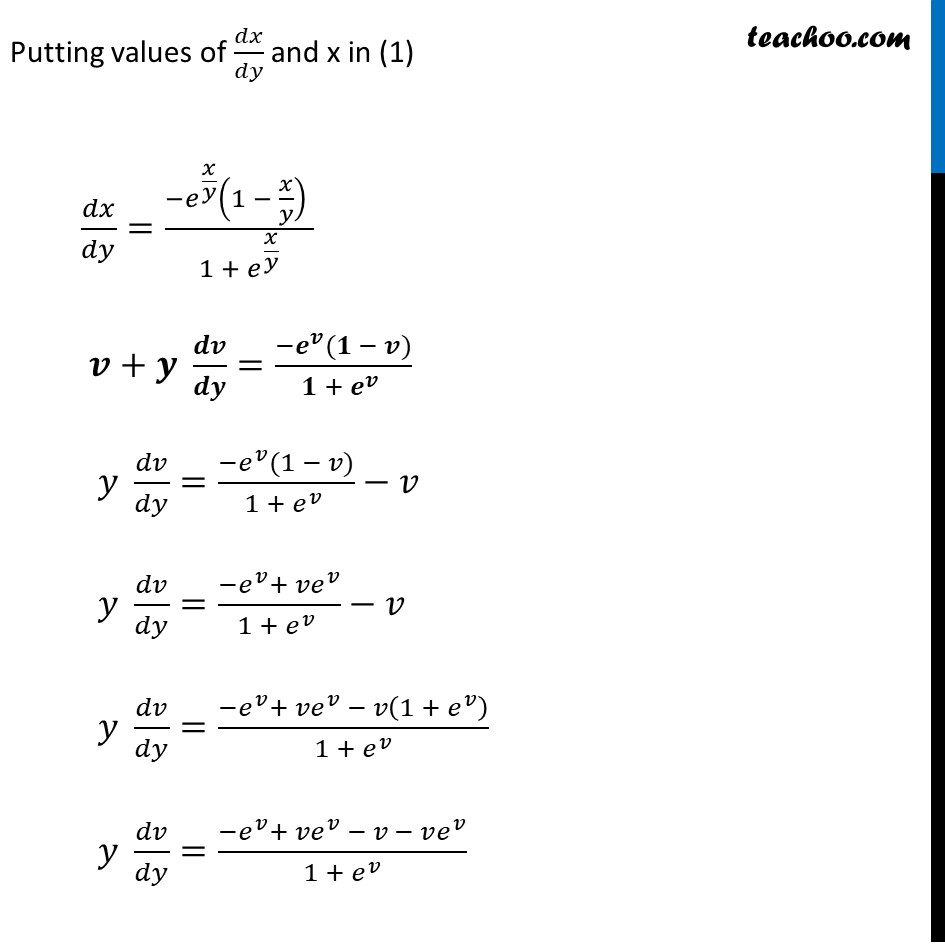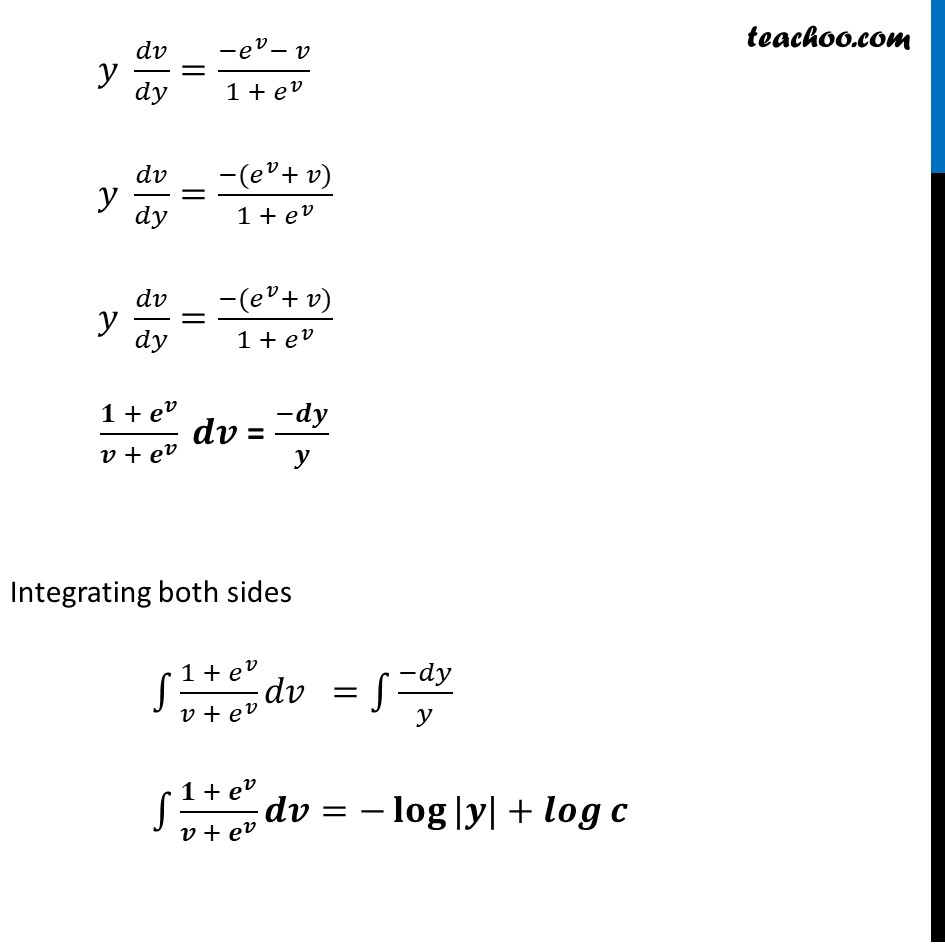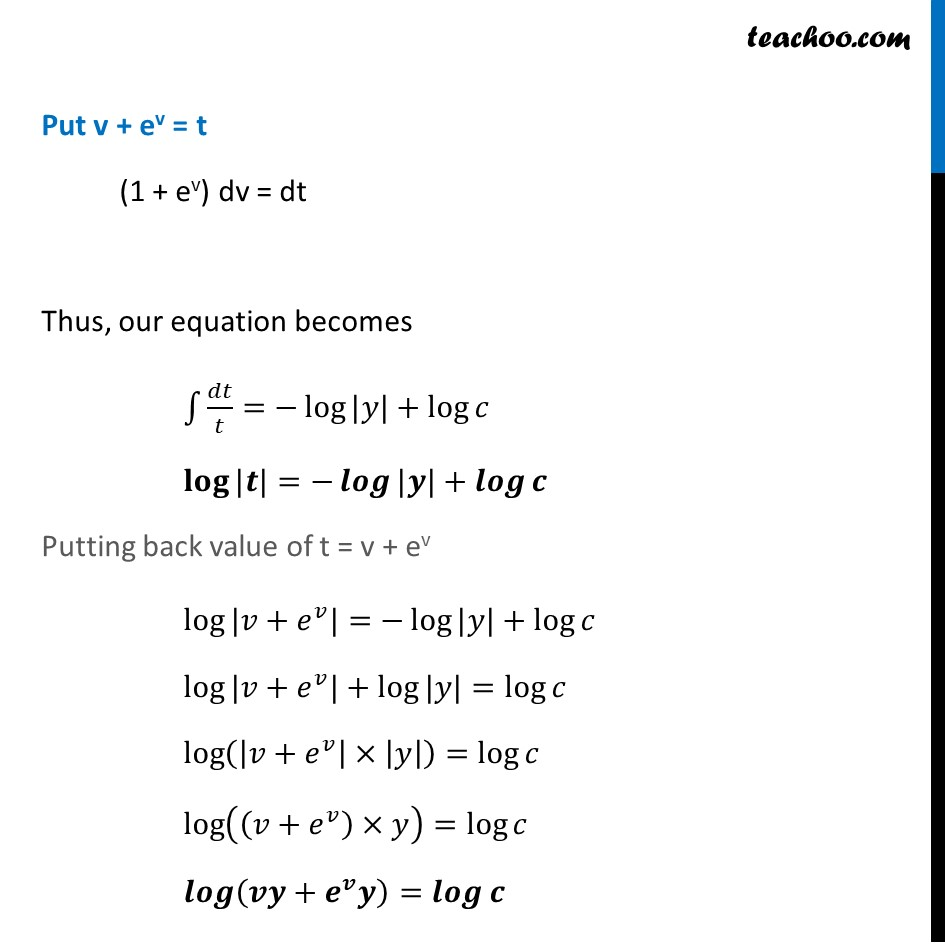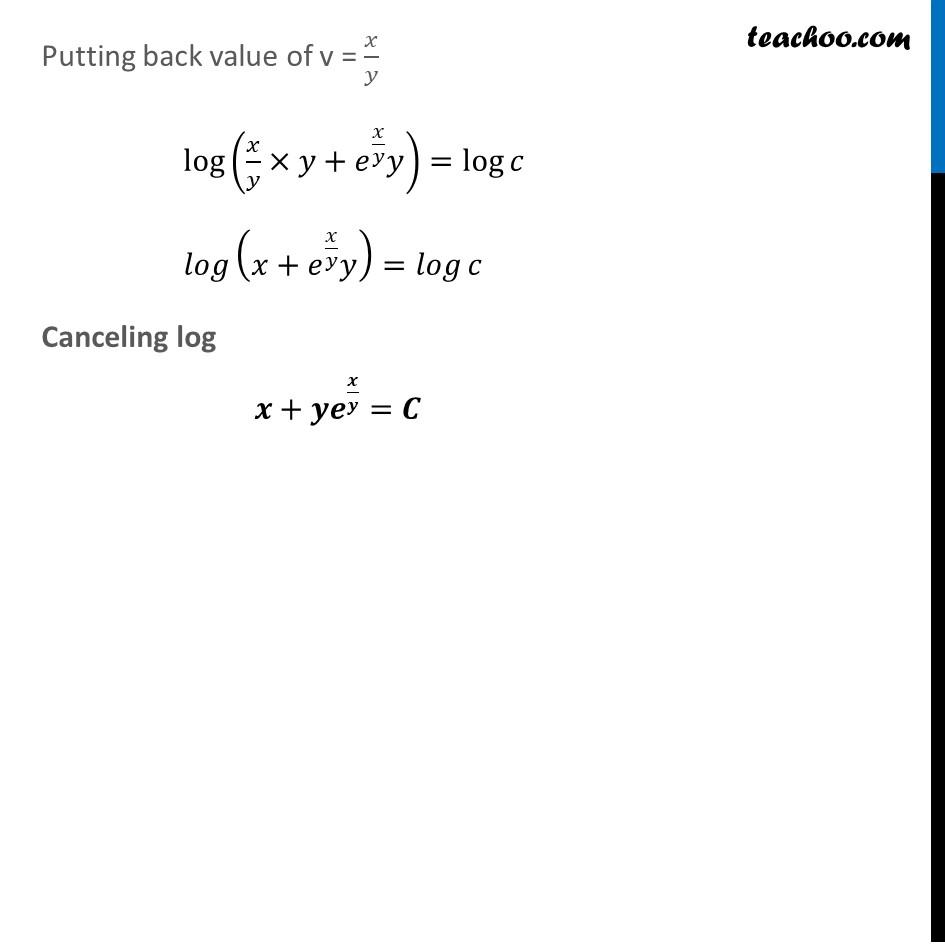Learn in your speed, with individual attention - Teachoo Maths 1-on-1 Class

### Transcript

Ex 9.4, 10 In each of the Exercise 1 to 10 , show that the given differential equation is homogeneous and solve each of them. (1+𝑒^(𝑥/𝑦) )𝑑𝑥+𝑒^(𝑥/𝑦) (1−𝑥/𝑦)𝑑𝑦=0 Step 1: Find 𝑑𝑥/𝑑𝑦 (1+𝑒^(𝑥/𝑦) )𝑑𝑥+𝑒^(𝑥/𝑦) (1−𝑥/𝑦)𝑑𝑦 = 0 (1+𝑒^(𝑥/𝑦) ) dx = −𝑒^(𝑥/𝑦) (1−𝑥/𝑦)𝑑𝑦 𝒅𝒙/𝒅𝒚 = (−𝒆^(𝒙/𝒚) (𝟏 − 𝒙/𝒚) )/(𝟏 + 𝒆^(𝒙/𝒚) ) Since the equation is in the form 𝑥/𝑦 , we will take 𝑑𝑥/𝑑𝑦 Instead of 𝑑𝑦/𝑑𝑥 Step 2: Put 𝑑𝑥/𝑑𝑦 = F(x, y) and find F(𝜆x, 𝜆y) F(x, y) = (−𝑒^(𝑥/𝑦) (1 − 𝑥/𝑦) )/(1 + 𝑒^(𝑥/𝑦) ) F(𝜆x, 𝜆y) = (−𝑒^(𝜆𝑥/𝜆𝑦) (1 − 𝜆𝑥/𝜆𝑦) )/(1 + 𝑒^(𝜆𝑥/𝜆𝑦) ) = (−𝑒^(𝑥/𝑦) (1 − 𝑥/𝑦) )/(1 + 𝑒^(𝑥/𝑦) ) = 𝐹(𝑥, 𝑦) Thus, F(x, y) is a homogenous equation function of order zero Therefore 𝑑𝑦/𝑑𝑥 is a homogenous differential equation Step 3: Solving 𝑑𝑥/𝑑𝑦 by putting 𝑥=𝑣𝑦 𝑑𝑥/𝑑𝑦= (−𝑒^(𝑥/𝑦) (1 − 𝑥/𝑦) )/(1 + 𝑒^(𝑥/𝑦) ) Put 𝒙=𝒗𝒚 Diff. w.r.t. 𝑦 𝑑𝑥/𝑑𝑦=𝑑/𝑑𝑦 (𝑣𝑦) 𝑑𝑥/𝑑𝑦=𝑦 . 𝑑𝑣/𝑑𝑦+𝑣 𝑑𝑦/𝑑𝑦 𝒅𝒙/𝒅𝒚=𝒚 . 𝒅𝒗/𝒅𝒚+𝒗 Putting values of 𝑑𝑥/𝑑𝑦 and x in (1) 𝑑𝑥/𝑑𝑦=(−𝑒^(𝑥/𝑦) (1 − 𝑥/𝑦) )/(1 + 𝑒^(𝑥/𝑦) ) 𝒗+𝒚 𝒅𝒗/𝒅𝒚=(−𝒆^𝒗 (𝟏 − 𝒗))/(𝟏 + 𝒆^𝒗 ) 𝑦 𝑑𝑣/𝑑𝑦=(−𝑒^𝑣 (1 − 𝑣))/(1 + 𝑒^𝑣 )−𝑣 𝑦 𝑑𝑣/𝑑𝑦=(−𝑒^𝑣+ 𝑣𝑒^𝑣)/(1 + 𝑒^𝑣 )−𝑣 𝑦 𝑑𝑣/𝑑𝑦=(−𝑒^𝑣+ 𝑣𝑒^𝑣 − 𝑣(1 + 𝑒^𝑣 ))/(1 + 𝑒^𝑣 ) 𝑦 𝑑𝑣/𝑑𝑦=(−𝑒^𝑣+ 𝑣𝑒^𝑣 − 𝑣 − 𝑣𝑒^𝑣)/(1 + 𝑒^𝑣 ) 𝑦 𝑑𝑣/𝑑𝑦=(−𝑒^𝑣− 𝑣)/(1 + 𝑒^𝑣 ) 𝑦 𝑑𝑣/𝑑𝑦=(−(𝑒^𝑣+ 𝑣))/(1 + 𝑒^𝑣 ) 𝑦 𝑑𝑣/𝑑𝑦=(−(𝑒^𝑣+ 𝑣))/(1 + 𝑒^𝑣 ) 〖𝟏 + 𝒆〗^𝒗/(𝒗 + 𝒆^𝒗 ) 𝒅𝒗 = (−𝒅𝒚)/𝒚 Integrating both sides ∫1▒〖〖1 + 𝑒〗^𝑣/(𝑣 + 𝑒^𝑣 ) 𝑑𝑣" " 〗 =∫1▒(−𝑑𝑦)/𝑦 ∫1▒〖〖𝟏 + 𝒆〗^𝒗/(𝒗 + 𝒆^𝒗 ) 𝒅𝒗〗=−𝐥𝐨𝐠⁡〖|𝒚|〗+𝒍𝒐𝒈⁡𝒄 Put v + ev = t (1 + ev) dv = dt Thus, our equation becomes ∫1▒𝑑𝑡/𝑡=−log⁡〖|𝑦|〗+log⁡𝑐 𝐥𝐨𝐠⁡〖|𝒕|〗=−𝒍𝒐𝒈⁡〖|𝒚|〗+𝒍𝒐𝒈⁡𝒄 Putting back value of t = v + ev log⁡〖|𝑣+𝑒^𝑣 |〗=−log⁡〖|𝑦|〗+log⁡𝑐 log⁡〖|𝑣+𝑒^𝑣 |〗+log⁡〖|𝑦|〗=log⁡𝑐 log⁡(|𝑣+𝑒^𝑣 |×|𝑦|)=log⁡𝑐 log⁡((𝑣+𝑒^𝑣 )×𝑦)=log⁡𝑐 𝒍𝒐𝒈⁡(𝒗𝒚+𝒆^𝒗 𝒚)=𝒍𝒐𝒈⁡𝒄 Putting back value of v = 𝑥/𝑦 log⁡(𝑥/𝑦×𝑦+𝑒^(𝑥/𝑦) 𝑦)=log⁡𝑐 𝑙𝑜𝑔⁡(𝑥+𝑒^(𝑥/𝑦) 𝑦)=𝑙𝑜𝑔⁡𝑐 Canceling log 𝒙+𝒚𝒆^(𝒙/𝒚)=𝑪